﻿ 基于离散元模型的冰阻力数值研究
 舰船科学技术2020, Vol. 42Issue (6): 14-19    DOI: 10.3404/j.issn.1672-7649.2020.06.003PDF

Numerical simulation on ice resistance using the discrete element method
GANG Xu-hao, TIAN Yu-kui, JI Shao-peng, KOU Ying
China Ship Scientific Research Center, Wuxi 214082, China
Abstract: In order to research the method of ship-ice resistance prediction, the discrete element method is used to simulate the ice resistance of the ship navigating in the level ice regions. The hull structure is constructed by triangular mesh, and the discrete element model of sea ice is constructed by using spherical elements, three-dimensional extended disks and extended polyhedral elements with the characteristic of cohesive fragmentation, and the effect of buoyance and drag force are considered. Based on the discrete element method, the paper calculates the total ice resistance of ship model;analyzed the effects of sea ice thickness, bending strength and ship speed on ice resistance. Also the results of ice resistance are compared with Lindqvist models. The research results can provide reference for ice resistance prediction and preliminary design optimization of ice-going ships.
Key words: ice-going ships     ice resistance     discrete element model     empirical model
0 引　言

1 海冰和船体结构的离散元方法 1.1 海冰的离散元方法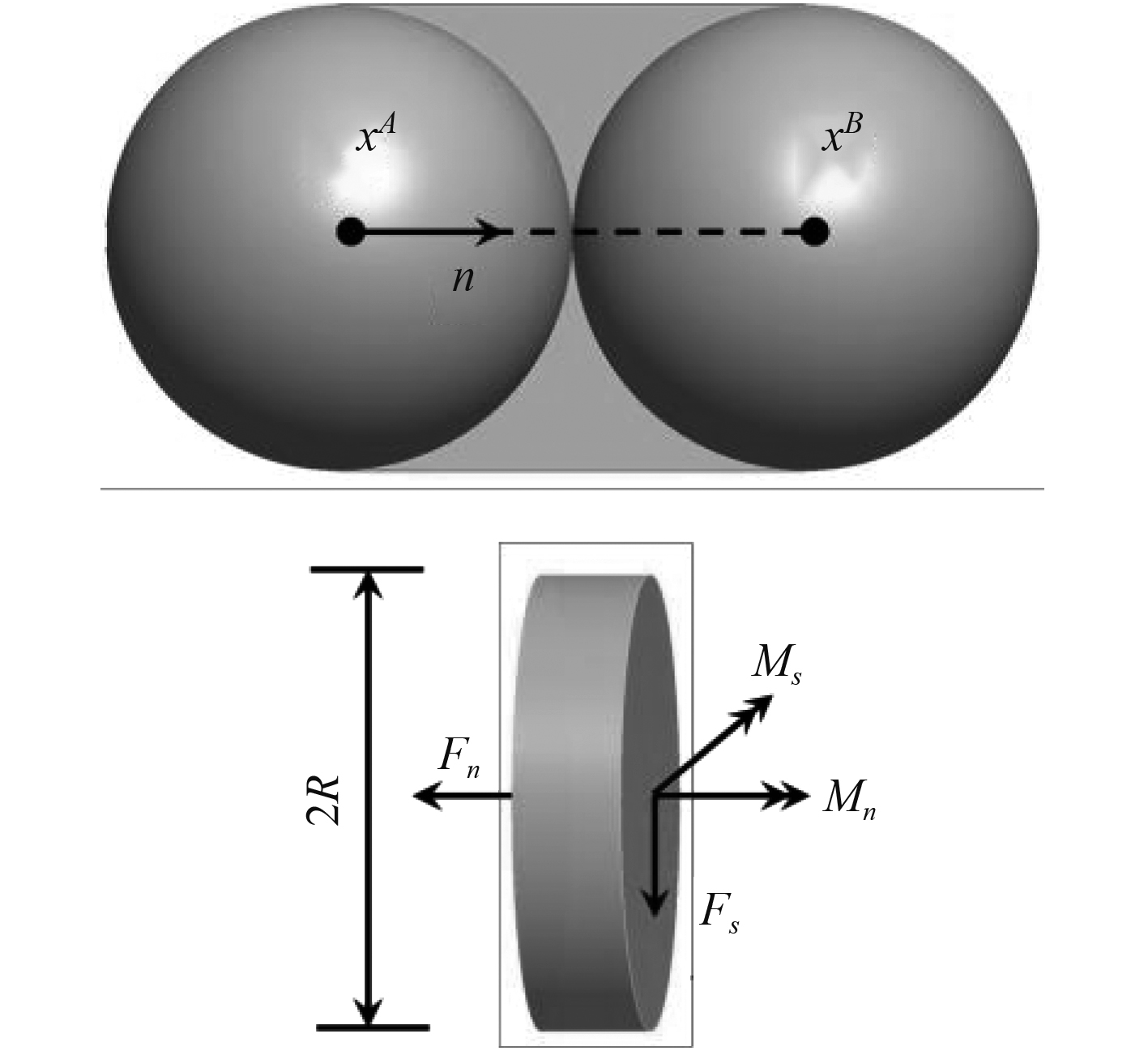图 1 颗粒之间的平行粘结模式 Fig. 1 Parallel bonding mode between particles
 ${\sigma _{\rm max}} = \frac{{ - {F_n}}}{A} + \frac{{\left| {{M_s}} \right|}}{I}R\text{，}$ (1)
 ${\tau _{\rm max}} = \frac{{{F_s}}}{A} + \frac{{\left| {{M_n}} \right|}}{J}R\text{。}$ (2)

1.2 船体的离散元方法表 1 船模船型参数 Tab.1 Ship type parameters of ship model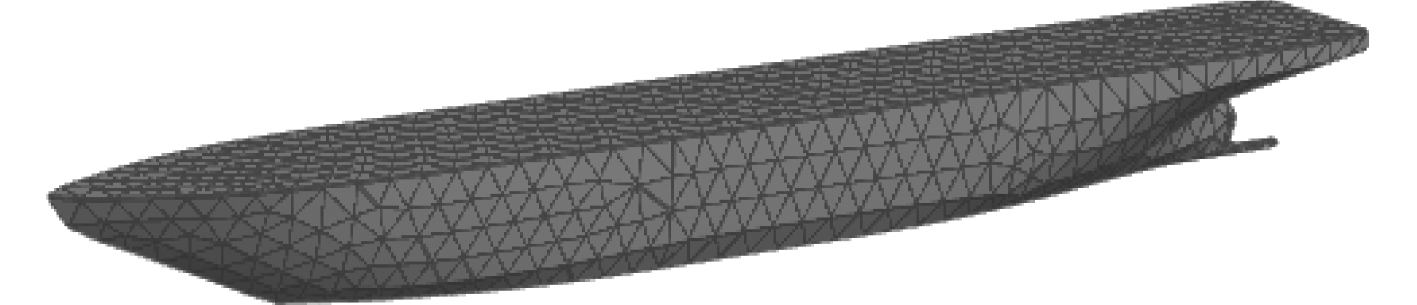图 2 船体的离散元方法 Fig. 2 The discrete element model of ship
2 船体在平整冰中航行的离散元分析表 2 离散元模拟的主要参数 Tab.2 Main parameters of discrete element simulation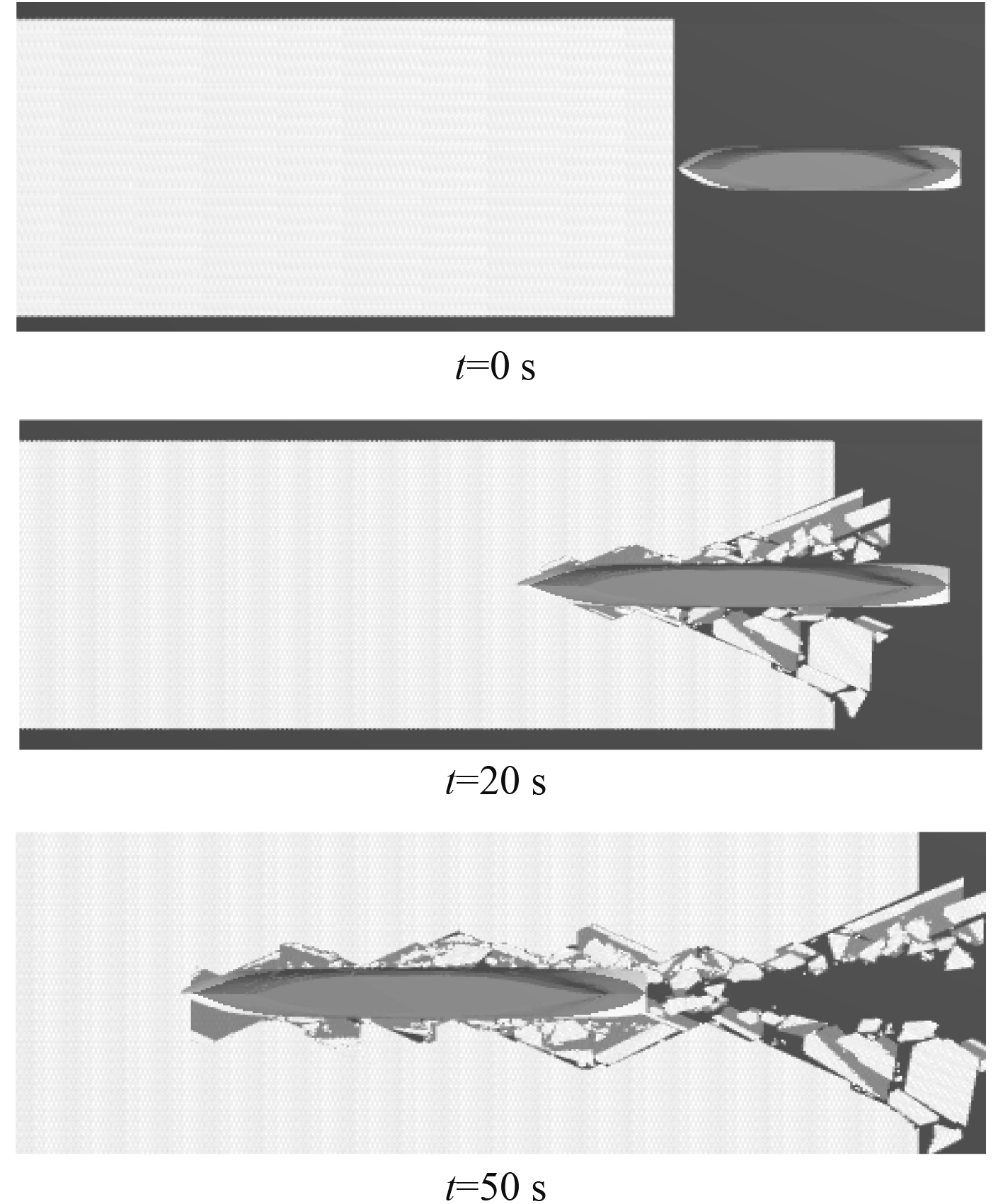图 3 船体在平整冰中航行的过程 Fig. 3 Process of hull sailing through flat ice表 3 冰厚变化时的计算结果 Tab.3 Calculation results when thickness changes表 4 弯曲强度变化时的计算结果 Tab.4 calculation results when flexural strength changes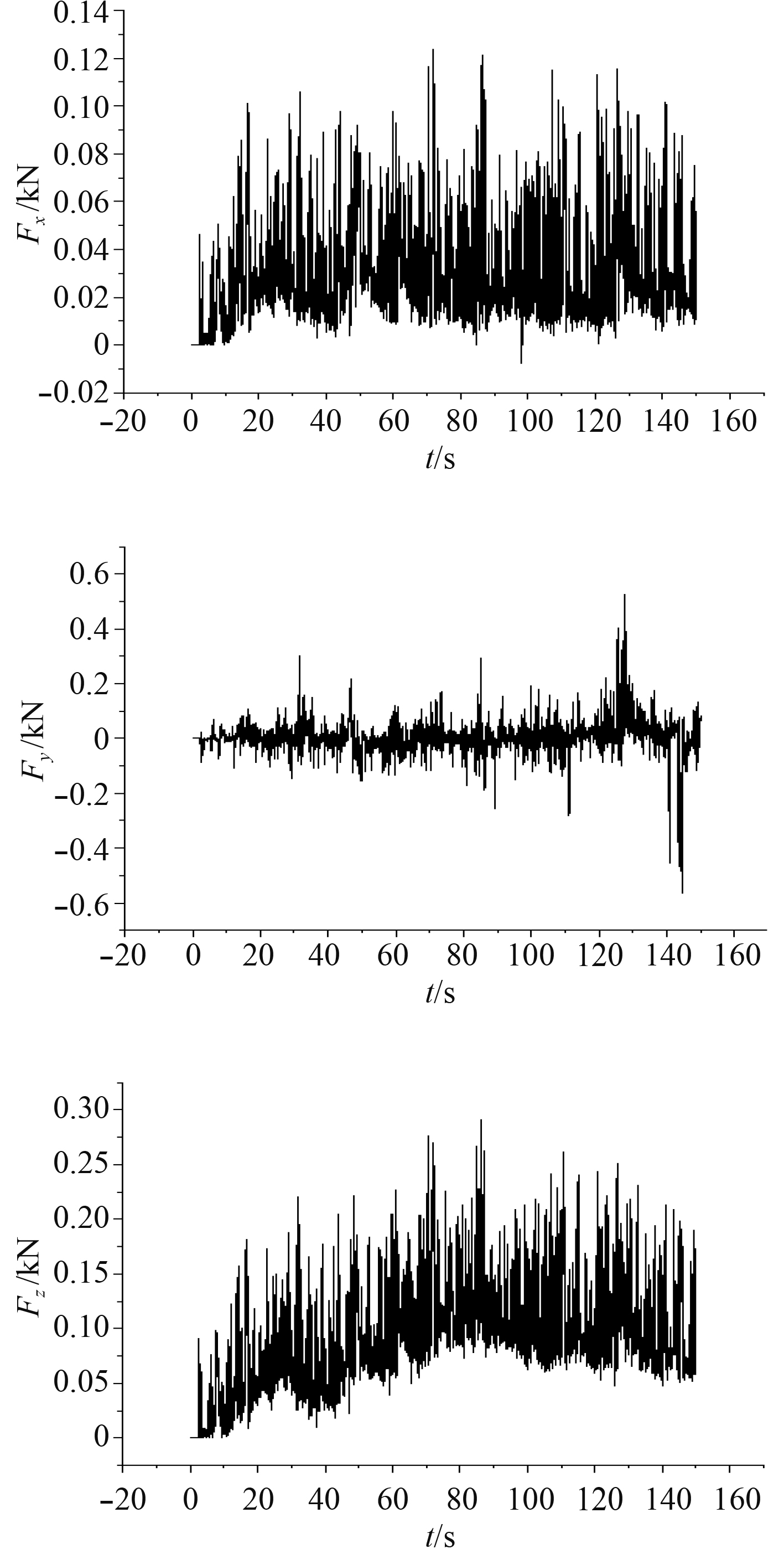图 4 X，Y，Z方向冰阻力曲线 Fig. 4 Ice resistance curve in X direction, Y directionand Z direction
2.1 航速对冰阻力的影响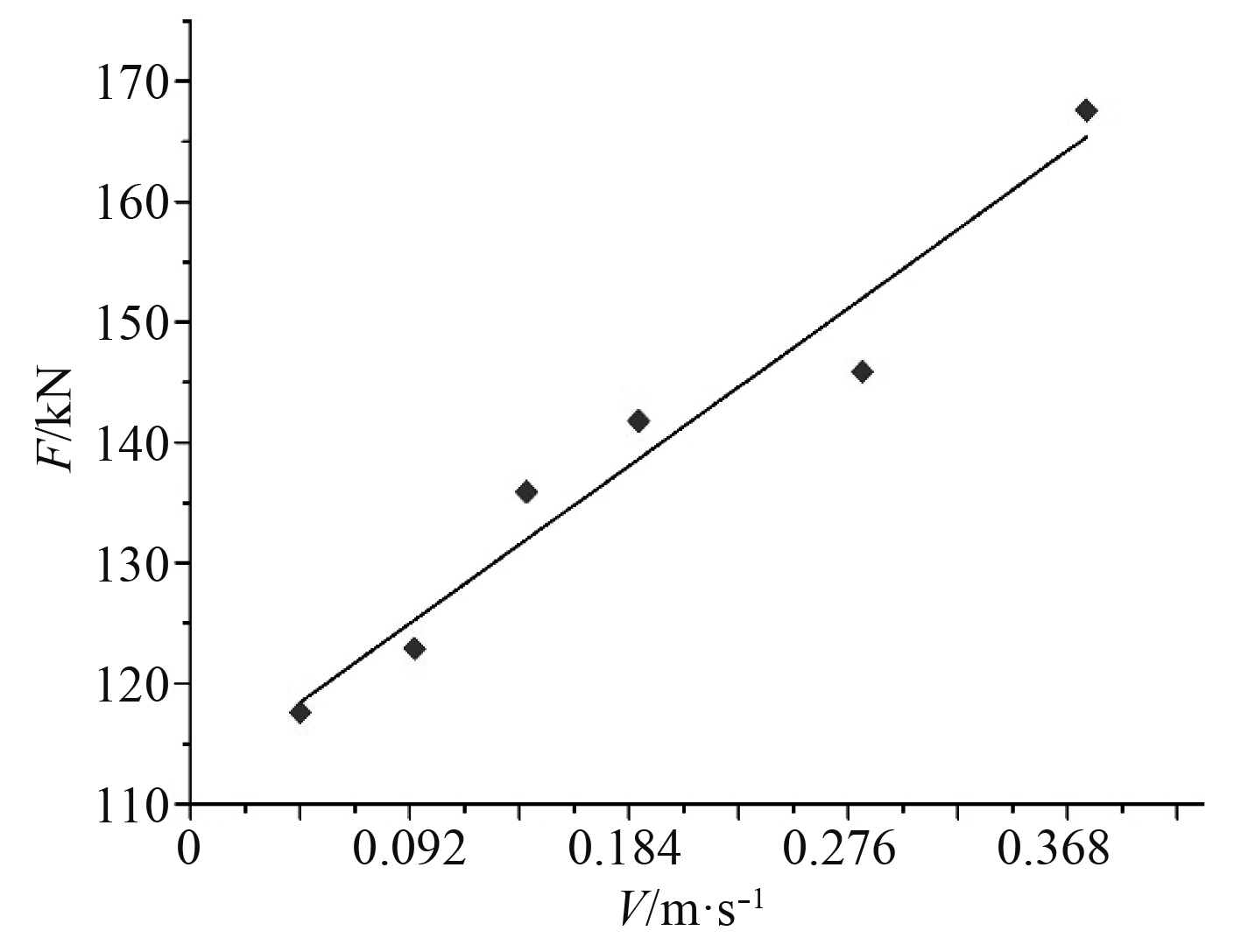图 5 冰阻力随航速的变化 Fig. 5 Variation of ice resistance with speed

2.2 冰厚对冰阻力的影响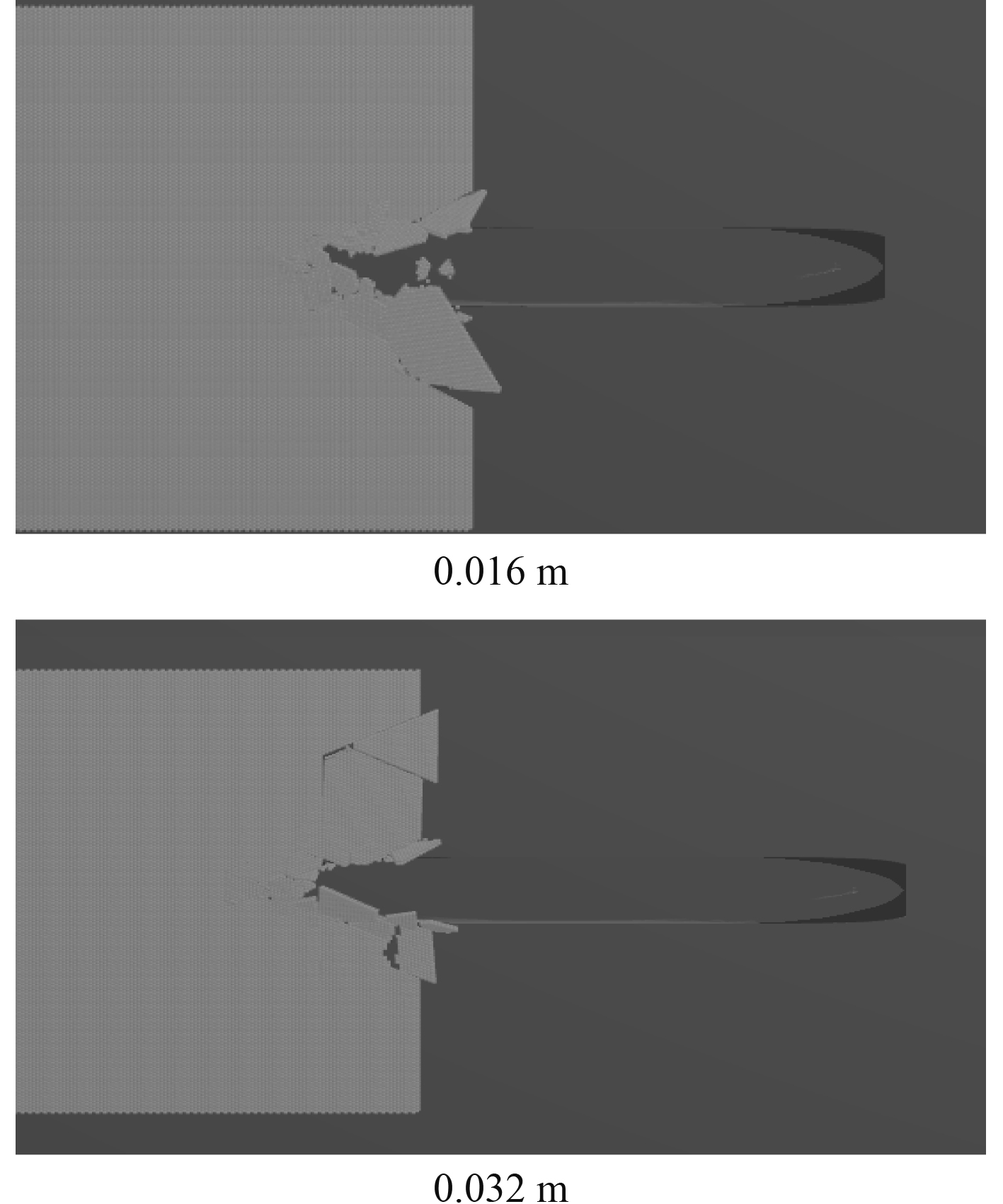图 6 在不同冰厚情况下的破碎形式 Fig. 6 Crushing forms under different ice thickness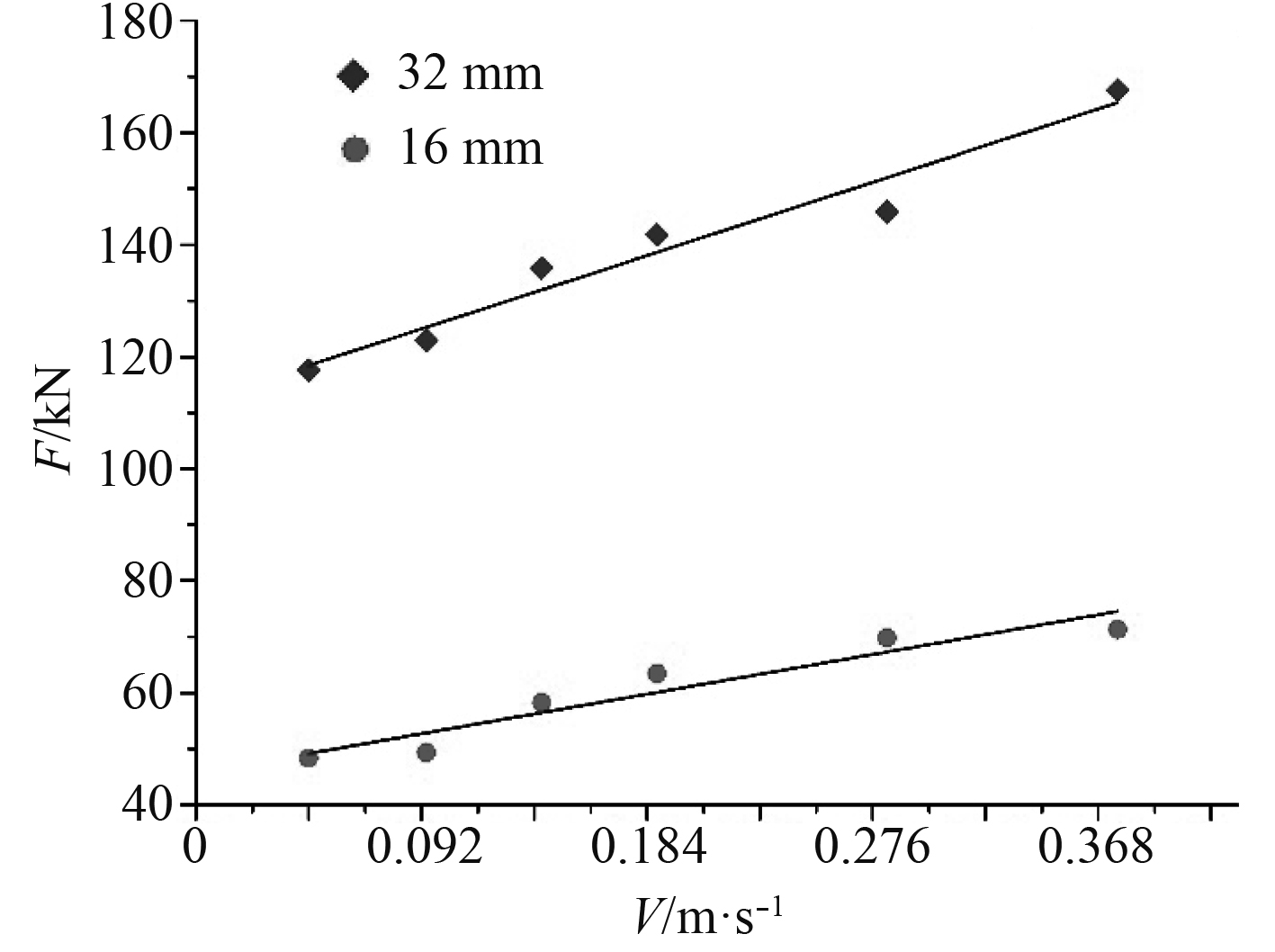图 7 冰阻力随冰厚的变化 Fig. 7 variation of ice resistance with ice thickness

2.3 弯曲强度对冰阻力的影响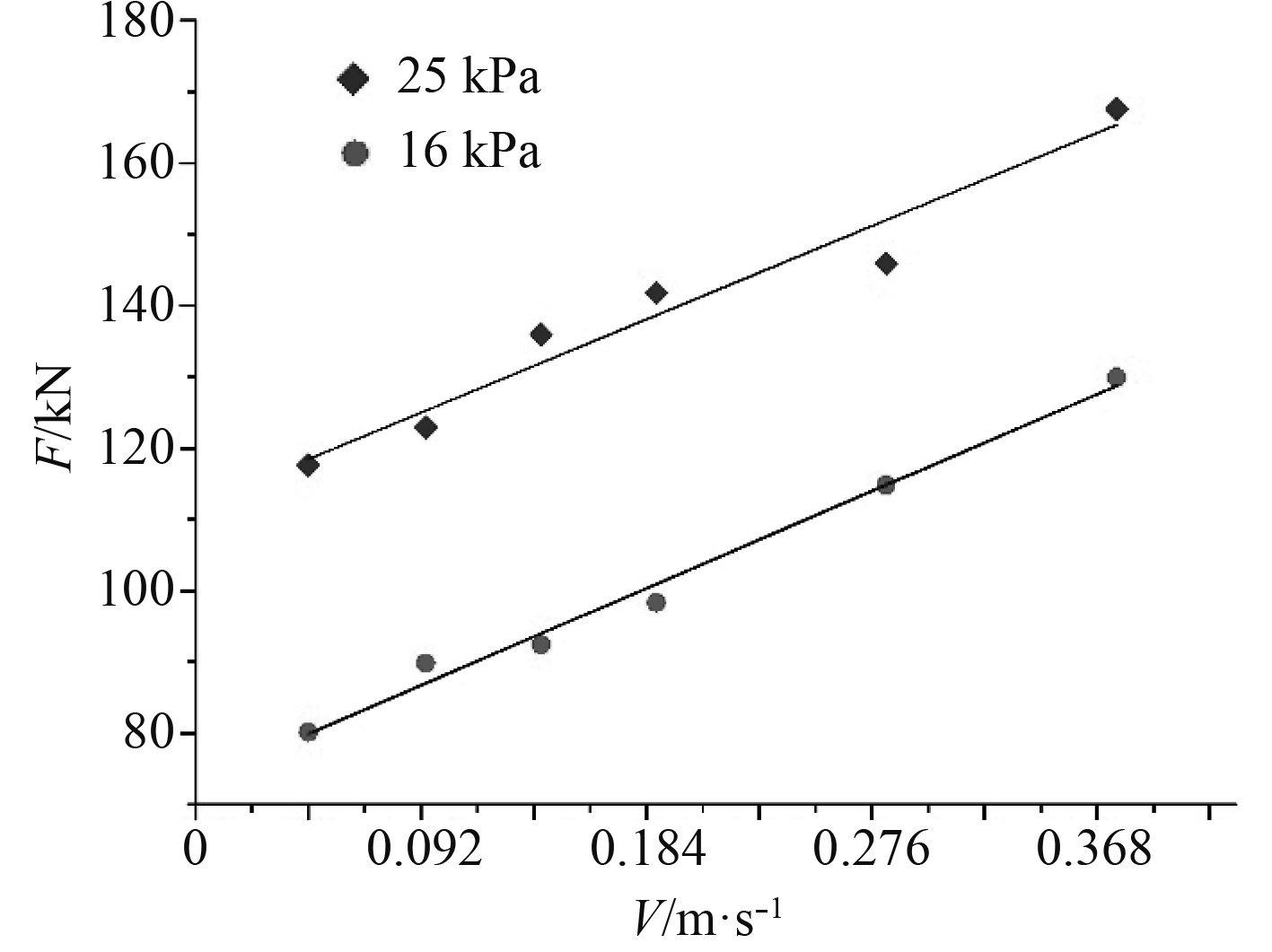图 8 冰阻力随弯曲强度的变化 Fig. 8 variation of ice resistance with flexural strength
3 模拟计算结果与Lindqvist经验模型结果对比

Lindqvist公式是根据在波罗的海波的尼亚湾进行的实船实验总结出来的经验模型。该模型阐述了一种简单的方式来预报船舶的冰阻力，能够快速对冰阻力进行简单的预报。

3.1 Lindqvist模型介绍

Lindqvis模型将冰阻力分解为挤压冰阻力、破冰阻力和浸没阻力3部分，并给出了与船舶主尺度、船型角度、速度、冰厚、摩擦系数及弯曲强度等参数相关的冰阻力表达式。Lindqvist经验公式表达式为：

 ${R}_{i}\left(v\right)=\left({R}_{c}+{R}_{b}\right)\left(1+1.4\frac{v}{\sqrt{gh}}\right)+{R}_{s}(1+9.4\frac{v}{\sqrt{gL}})\text{，}$ (3)
 ${R}_{c}=0.5{\sigma }_{b}{h}^{2}\frac{{\rm tan}\varphi +\mu {\rm cos}\varphi /{\rm cos}\psi }{1-\mu {\rm sin}\varphi /{\rm cos}\psi } \text{，}$ (4)
 ${R}_{b}=\frac{27}{64}{\sigma }_{b}B\frac{{h}^{1.5}}{\sqrt{\frac{E}{12\left(1-{\nu }^{2}\right)}}}\frac{{\rm tan}\psi +\mu {\rm cos}\varphi }{{\rm cos}\varphi {\rm sin}\alpha }(1+\frac{1}{{\rm cos}\psi }) \text{，}$ (5)
 $\begin{split} {R_s} = & \left( {{\rho _w} - {\rho _i}} \right)ghB\left( {T\frac{{B + T}}{{B + 2T}} + \mu \left( {0.7L - \frac{T}{{{\text{tan}}\varphi }} - } \right.} \right. \\ & \left. {\left. {\frac{B}{{4{\text{tan}}\alpha }} + T{\text{cos}}\varphi {\text{cos}}\psi \sqrt {\frac{1}{{{\text{sin}}{\varphi ^2}}} + \frac{1}{{{\text{tan}}{\alpha ^2}}}} } \right)} \right] \end{split}\text{。}$ (5)

3.2 数值计算结果和经验模型结果的比较表 5 冰厚变化时的计算结果对比 Tab.5 Comparison of result when ice thickness changes表 6 弯曲强度变化时的计算结果对比 Tab.6 Comparison of result when flexural strength changes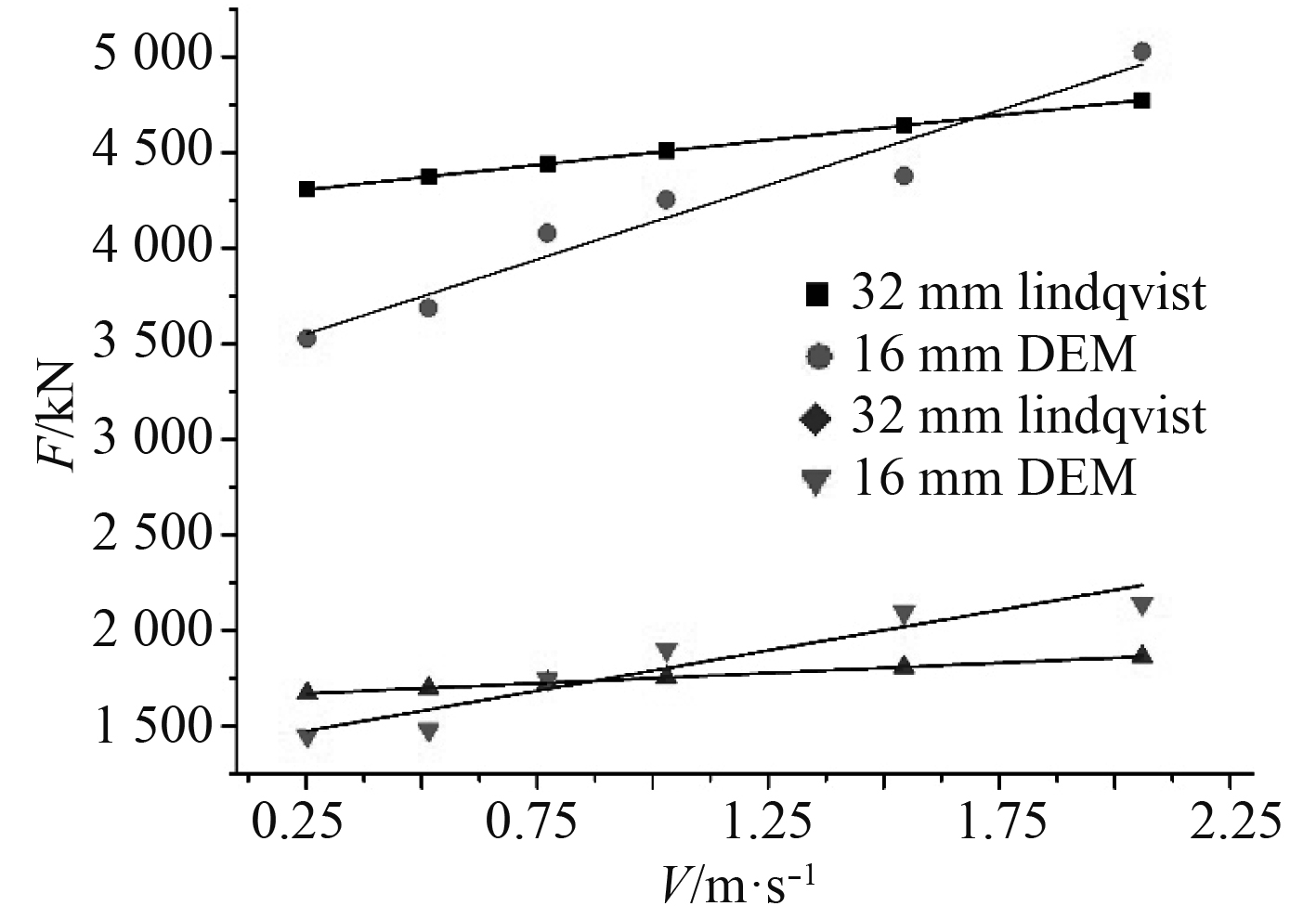图 9 冰厚变化时的计算结果 Fig. 9 Calculation results when thickness changes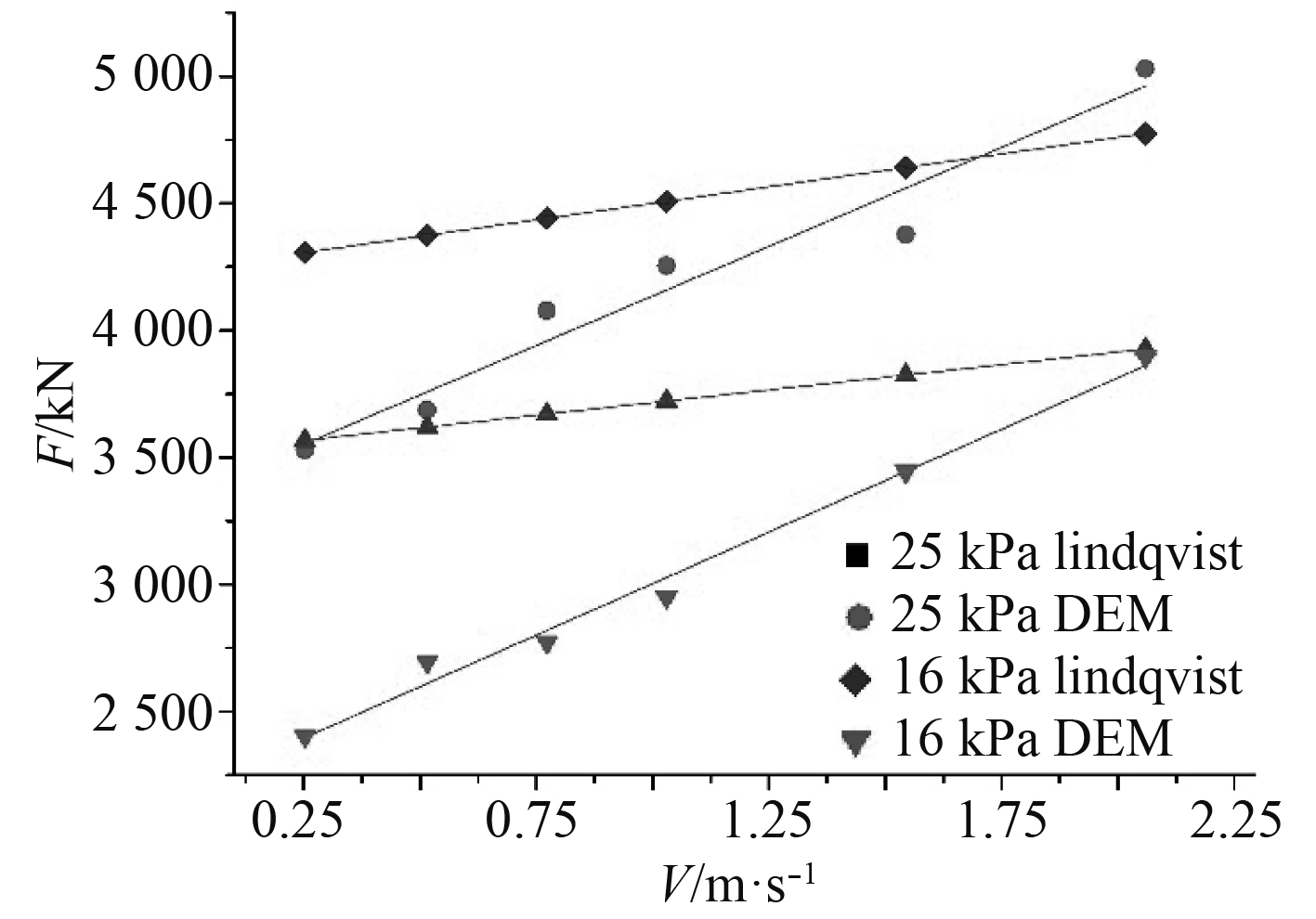图 10 弯曲强度变化时的计算结果 Fig. 10 Calculation results when flexural strength changes

4 结　语

  龚榆峰, 张正艺, 刘敬喜, 等. 基于ABAQUS的海冰单元开发及冰载荷直接计算法[J]. 中国舰船研究, 2017, 12(3): 75-85. GONG Y F, ZHANG Z Y, LIU J X, et al. Development of sea ice element and direct analysis method of predicting ice loads based on ABAQUS[J]. Chinese Journal of Ship Research, 2017, 12(3): 75-85. DOI:10.3969/j.issn.1673-3185.2017.03.011  吴炜. 考虑不同漂角的船舶冰阻力模型试验研究[D]. 天津: 天津大学, 2019, 11. WU wei. Model tests on ice resistance of an icebreaking ships with different ice drift angles[D]. Tianjin: Tianjin Univercity, 2016, 11.  狄少丞, 季顺迎, 薛彦卓. 船舶在平整冰行进过程的离散元分析[J]. 海样工程, 2017, 35(3): 59-69. DI S C, JI S Y, XUE Y Z. Discrete element analysis of ship moving on flat ice[J]. The Ocean Engineering, 2017, 35(3): 59-69.  GAGNON R, CUMMING D, RITCH R, et al. Overview accompaniment for papers on the barge bit impact trials[J]. Cold Regions Science and Technology, 2008(52): 1-6.  RALPH F, MCKENNA R, GAGNON R. Iceberg characterization for the barge bit impact study[J]. Cold Regions Science and Technology, 2008(52): 7-28.  HOPKINS M A. Onshore ice pile-up: a comparison between experiments and simulations[J]. ColdRegions Science and Technology, 1997, 26(3): 205-214.  SHEN H H, HIBLER W D., LEPPARANTA M.. The role of floe collisions in sea ice rheology[J]. Journal of Geophysical Research, 1987, 92(C10): 7085-709.  YUE Q, BI X. Ice-induced jacket structure vibrations in Bohai Sea[J]. Journal of Cold Regions Engineering, 2000, 14(2): 81-92. DOI:10.1061/(ASCE)0887-381X(2000)14:2(81)  季顺迎, 王安良, 车啸飞, 等. 椎体导管架海洋平台冰激结构振动响应分析[J]. 海洋工程, 2011, 29(2): 32-38. JI S Y, WANG A L, CHE X F, et al. Vibration response analysis of ice induced structures on cone jacket platform[J]. The Ocean Engineering, 2011, 29(2): 32-38. DOI:10.3969/j.issn.1005-9865.2011.02.005  季顺迎, 狄少丞, 李正, 等. 海冰与直立结构相互作用的离散单元数值模拟[J]. 工程力学, 2013, 30(1): 463-469. JI S Y, DI S C, LI Z, et al. Discrete element numerical simulation of interaction between sea ice and vertical structures[J]. Engineering Mechanicals, 2013, 30(1): 463-469.  JI shum Ying. Discrete element model and ice load analysis for ship sailing in broken ice area[J]. Chinese Journal of Theoretical and Applied Mechanics, 2013, 45(6): 868-877.  JI S Y, DI S C, LONG X. DEM simulation of uniaxial compressive and flexural strength of sea ice: parametric study[J]. Journal of Engineering Mechanics, 2016.  JI S, SUN, S, LONG, X, et al. Discrete element modeling of ice loads on ship and offshore structures[C]//The 7th International Conference on Discrete Element Methods, Dalian, China 2016.  LINDQVIST G. A straightforward method for calculation of ice resistance of ships[C]//Proceedings of the Tenth International Conference on Port and Ocean Engineering under Arctic Conditions. Lulea, Sweden, 1989: 722-735.